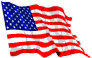Deliberate PracticeE-mail me now: Mr. Hansen

The dates shown indicate the date the assignments were given.
Unless otherwise indicated they are due on the first non-test school day following their assignment (usually, the next day).

1st Trimester

As long as we are homebound you will take photographs homework and attach them to the
blank assignment page provided in Google Classroom (GC), daily           Don't procrastinate!

Trimester 1

Week of 10/19 Click the link to see the plan: Link to Work

Week of 10/12 Click the link to see the plan: Link to Work

Week of 10/5 Click the link to see the plan:  Link to Work

Week of 9/28 Click the link to see the plan:  Link to Work

Week of 9/21 Click the link to see the plan:  Link to Work

Week of 9/14 Click the link to see the plan:  Link to Work

09/08 Tuesday:  Mini-Lesson (ML): Arithmetic Powers
Vocab: Arithmetic, power, base, exponent
Notes: Cognate question: What does 3^4 mean?
Q: What does simplify mean?
A: Do all operations possible
Quick Credit: (QC): A7 QC1

Lesson: Intro to Algebra (what is Algebra?)
Solve algebraic equations mentally
Translate English-to-Algebra equations on lapboard and solve
Algebraic powers
HW: 2- KTA3 and 3-KTA3, all questions

09/09 Wednesday  Lesson: Intro to Algebra (what is Algebra?)
Solve algebraic equations mentally
Translate English-to-Algebra equations on lapboard and solve
Algebraic powers
HW: 2- KTA3 and 3-KTA3, all questions
Wednesday  ML: Differentiate between -2^3 and (-2)^3
Notes: Q: What is the name of this expression: -2^3
A: The opposite of 2 to the third power
Q: What is the name of this expression: (-2)^3
A: Negative 2 to the third power
QC: A7-QC2

Lesson: Use exponents to shorten an expression; Write out an expression the long way.
8-KTA2 and 9-KTA2
HW: 13-KTA2 #2-32 evens

09/10 Thursday  ML: Zero exponent
Vocab: reciprocal
Notes: Cognate question: What does it mean to say that two
Numbers or quantities are reciprocals of one another?
Answer: That their product is one
Q: How do we read this expression? 4^-3
A: The reciprocal of 4 to the third power.
Q: What is the reciprocal of 4 to the 3rd power?
A: One over 4 to the third power.
QC: A7-QC3

Lesson: Multiply algebraic monomials (exponents are positive integers)
14-KTA2, odds
HW: 14-KTA2, #2-24 evens

09/11 Friday  ML: Powers overview (Arithmetic powers with positive, negative and zero exponents; bases positive or negative)
QC: A7-QC4
Lesson: Review for test (material covered: all cognates, questions, definitions; solve linear equations mentally; multiply algebraic monomials)
QUIZ: Quiz 1